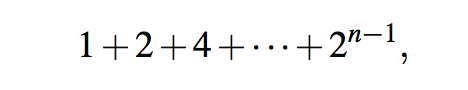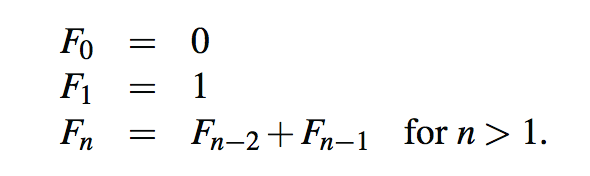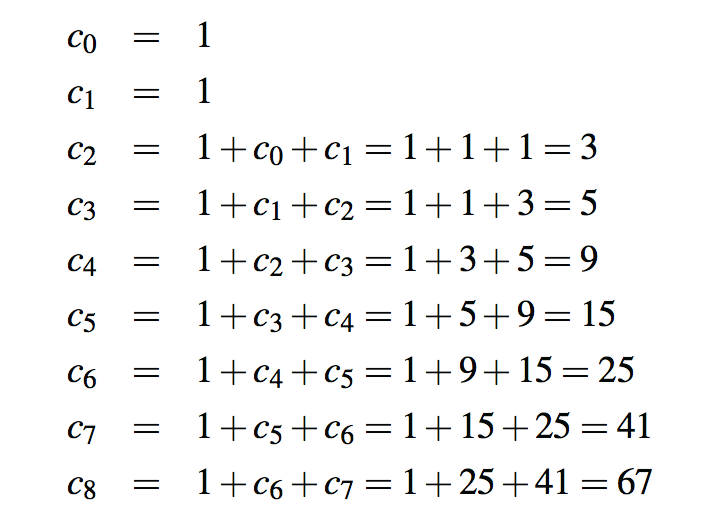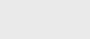3年前 (2020-10-18) |   抢沙发  461

[收起] 文章目录

## 递归的缺点和问题

``````/∗∗ Returns true if there are no duplicate values from data[low] through data[high].∗/
public static boolean unique3(int[ ] data, int low, int high) {
if (low >= high)
return true; // at most one item
else if (!unique3(data, low, high−1))
return false; // duplicate in first n−1
else if (!unique3(data, low+1, high))
return false; // duplicate in last n−1
else
return (data[low] != data[high]); // do first and last differ?
}``````### 计算Fibonacci数的一种低效递归算法``````/∗∗ Returns the nth Fibonacci number (inefficiently). ∗/
public static long fibonacciBad(int n) {
if (n <= 1)
return n;
else
}``````### 计算Fibonacci数的一种有效递归方法

``````/∗∗ Returns array containing the pair of Fibonacci numbers, F(n) and F(n−1). ∗/
public static long[ ] fibonacciGood(int n) {
if (n <= 1) {
long[ ] answer = {n, 0};
} else {
long[ ] temp = fibonacciGood(n − 1); // returns {Fn−1, Fn−2}
long[ ] answer = {temp + temp, temp}; // we want {Fn, Fn−1}
}
}``````

### Java中的最大递归深度

``````/∗∗ Don't call this (infinite) version. ∗/
public static int fibonacci(int n) {
return fibonacci(n); // After all Fn does equal Fn
}``````

``return binarySearch(data, target, mid, high); // sending mid, not mid+1``

## 消除尾递归

``return n ∗ factorial(n−1);``

``````/∗∗ Returns true if the target value is found in the data array. ∗/
public static boolean binarySearchIterative(int[ ] data, int target) {
int low = 0;
int high = data.length − 1;
while (low <= high) {
int mid = (low + high) / 2;
if (target == data[mid]) // found a match
return true;
else if (target < data[mid])
high = mid − 1; // only consider values left of mid
else
low = mid + 1; // only consider values right of mid
}
return false; // loop ended without success
}``````

``````/∗∗ Reverses the contents of the given array. ∗/
public static void reverseIterative(int[ ] data) {
int low = 0, high = data.length − 1;
while (low < high) { // swap data[low] and data[high]
int temp = data[low];
data[low++] = data[high]; // post-increment of low
data[high−−] = temp; // post-decrement of high
}
}``````### 注册